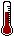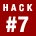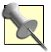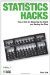# Hack 7. Measure UpFour levels of measurement determine how the scores produced in measurement can be used. If you have not measured at the right level, you might not be able to play with those scores the way you want.

Statistical procedures analyze numbers. The numbers must have meaning, of course; otherwise, the exercises are of little value. Statisticians call numbers with meaning scores. Not all the scores used in statistics, however, are created equal. Scores have different amounts of information in them, depending on the rules followed for creating the scores.

When you decide to measure something, you must choose the rules by which you assign scores very carefully. The level of measurement determines which sorts of statistical analyses are appropriate, which will work, and which will be meaningful.Measurement is the meaningful assignment of numbers to things. The things can be concrete objects, such as rocks, or abstract concepts, such as intelligence.

Here's an example of what I mean when I say not all scores are created equal. Imagine your five children took a spelling test. Chuck scored a 90, Dick and Jan got 80s, Bob scored 75, and Don got only 50 out of 100 correct. If a friend asked how your kids did on the big test, you might report that they averaged 75. This is a reasonable summary. Now, imagine that your five children ran a foot race against each other. Bob was first, Jan second, Dick third, Chuck fourth, and Don fifth. Your nosey friend again asks how they did. With a proud smile, you report that they averaged third place. This is not such a reasonable summary, because it provides no information. In both cases, though, scores were used to indicate performance. The difference lies only in the level of measurement used.

There are four levels of measurementthat is, four ways that numbers are used as scores. The levels differ in the amount of information provided and the types of mathematical and statistical analyses that can be meaningfully conducted on them. The four levels of measurement are nominal, ordinal, interval, and ratio.

#### Using Numbers as Labels

If you are planning to use scores to indicate only that the things belong to different groups, measure at the nominal level. The nominal level of measurement uses numbers only as names: labels for various categories (nominal means "in name only").

For example, a scientist who collects data on men and women, using a 1 to indicate a male subject and a 2 to indicate a female subject, is using the numbers at a nominal level. Notice that even though the number 2 is mathematically greater than the number 1, a 2 in this data set does not mean more of anything. It is used only as a name.

#### Using Numbers to Show Sequence

If you want to analyze your scores in ways that rely on performance measured as evidence of some sequence or order, measure at the ordinal level. Ordinal measurement provides all the information the nominal level provides, but it adds information about the order of the scores. Numbers with greater values can be compared with numbers at lower values, and the people or otters or whatever was measured can be placed into a meaningful order.

Take, for example, your rank order in your high school class. The valedictorian is usually the person who received a score of 1 when grade point averages are compared. Notice that you can compare scores to each other, but you don't know anything about the distance between the scores. In a footrace, the first-place finisher might have been just a second ahead of the second-place runner, while the second-place runner might have been 30 seconds ahead of the runner who came in third place.

#### Using Numbers to Show Distance

Interval level measurement uses numbers in a way that provides all the information of earlier levels, but adds an element of precision. This level of measurement produces scores that are interpreted as having an equal difference between any two adjacent scores.

For example, on a Fahrenheit thermometer, the meaningful difference between 70 and 69 degrees1 degreeis equal to the difference between 32 and 31 degrees. That one degree is assumed to be the same amount of heat (or, if you prefer, pressure on the liquid in the thermometer), regardless of where on the scale the interval exists.

The interval level provides much more information than the ordinal level, and you can now meaningfully average scores. Most educational and psychological measurement takes place at the interval level.

Though interval level measurement would seem to solve all of our problems in terms of what we can and cannot do statistically, there are still some mathematical operations that are not meaningful at this level. For instance, we don't make comparisons using fractions or proportions. Think about the way we talk about temperature. If a 40-degree day follows an 80-degree day, we do not say, "It is half as hot today as yesterday." We also don't refer to a student with a 120 IQ as "one-third smarter" than a student with a 90 IQ.The word interval is a term from old-time castle architecture. You know those tall towers or turrets where archers were stationed for defense? Around the circular tops, there was typically a pattern of a protective stone, then a gap for launching arrows, followed by another protective stone, and so on. The gaps were called intervals ("between walls"), and the best designed defenses had the stones and gaps at equal intervals to provide 360-degree protection.

#### Using Numbers to Count in Concrete Ways

The highest level of measurement, ratio, provides all the information of the lower levels but also allows for proportional comparisons and the creation of percentages. Ratio level measurement is actually the most common and intuitive way in which we observe and take accounting of the natural world. When we count, we are at the ratio level. How many dogs are on your neighbor's porch? The answer is at the ratio level.

Ratio level measurement provides so much information and allows for all possible statistical manipulations because ratio scales use a true zero. A true zero means that a person could score 0 on the scale and really have zero of the characteristic being measured. Though a Fahrenheit temperature scale, for example, does have a zero on it, a zero-degree day does not mean there is absolutely no heat. On interval scales, such as in our thermometer example, scores can be negative numbers. At the ratio level of measurement, there are no negative numbers.

#### Choosing Your Level of Measurement

Which level of measurement is right for you? Because of the advantages of moving to at least the interval level, most social scientists prefer to measure at the interval or ratio level. At the interval level, you can safely produce descriptive statistics and conduct inferential statistical analyses, such as t tests, analyses of variance, and correlational analyses. Table 1-6 provides a summary of the strengths and weaknesses of each level of measurement.

##### Table Levels of measurement
Level of measurementStrengthWeakness
NominalDescribes categorical data.Numbers do not indicate quantity.
OrdinalAllows comparison between scores.Difficult to summarize scores.
IntervalMost statistical analyses are possible.Proportional comparisons are not possible.
RatioTrue zero allows for all possible statistical analyses.Some variables of interest do not have a true zero.

To choose the correct statistical analysis of data created by others, identify the level of measurement used and benefit from its strengths. If you are creating the data yourself, consider measuring up: using the highest level of measurement that you can.

#### Controversial Tools

Since the levels of measurement became commonly accepted in the 1950s, there has been some debate about whether we really need to clearly be at the interval level to conduct statistical analyses. There are many common forms of measurement (e.g., attitude scales, knowledge tests, or personality measures) that are not unequivocally at the interval level, but might be somewhere near the top of the ordinal level range. Can we safely use this level of data in analyses requiring interval scaling?

A majority consensus in the research literature is that if you are at least at the ordinal level and believe that you can make meaning out of interval-level statistical analyses, then you can safely perform inferential statistical analyses on this type of data. In the real world of research, by the way, almost everybody chooses this approach (whether they know it or not).

The basic value of making analytical decisions based on level of measurement is hard to deny, however. A classic example of the importance of measurement levels is described by Frederick Lord in his 1953 article "On the Statistical Treatment of Football Numbers" (American Psychologist, Vol. 8, 750-751). An absent-minded statistician eagerly analyzes some data given him concerning the college football team, and produces a report full of means and standard deviations and other sophisticated analyses. The data, though, turn out to be the numbers from the backs of the players' jerseys. A clear instance of not paying attention to level of measurement, perhaps, but the statistician stands by his report. The numbers themselves, he explains, don't know where they came from; they behave the same way regardless.Statistics Hacks: Tips & Tools for Measuring the World and Beating the Odds
ISBN: 0596101643
EAN: 2147483647
Year: 2004
Pages: 114
Authors: Bruce Frey

Similar book on Amazon